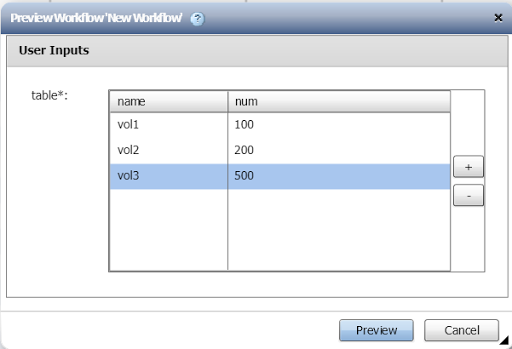## Wednesday, June 20, 2018

### How to calculate a sum in a repeat row in WFA

Ever needed to add a repeat row and calculate a sum on the fly ?
This is built into wfa already.

For example, you want a user input table, where you add qtrees and their sizes, but you would need a sum at some point so create the volume (where the size is the sum of the qtrees).

READ ALL THE WAY DOWN !
Just create a repeat row and add a custom variable (sum in my case) and have it calculate based on the table input (using row index and column index).
Also note I cast them to a number but multiplying with 1 (*1), a little trick I use to force to a number, as it would otherwise concatenate them as strings instead (example : 100 + 200 = 100200).#### 1. Index starts at 0, and gets added up in the end.

This means that the first time (row 1), your sum = 0.  And even though this is a classic for-loop, in this case you would expect it to be 100 in this example.  On the last row, sum would be 300, as 500 isn't added yet.  Only when the row ends, the 500 is added.  So that's a bummer !  The means if I ran the example above and I evaluate sum in every row, this would be : 0, 100, 300.  However, you would (naturally) expect : 100, 300, 800.

#### 2. Sum is evaluated on the go (not like constants upfront)

This means you can use sum after the repeat row, but NOT before !

### Summary

So, I you want your sum, you have 2 options.
Either use the above in an empty repeat row, and after the repeat row, you will have the result 800.

Or, if you want to use the sums in the row itself and still want the behavior of 100,300,800 (and not 0,100,300), then use this instead !The trick here is to set the intial value to the first item (100).  Then we use the same equation, but we use rowindex+1.  However, if we use rowindex+1, the last time, we will get an error (index ouf of range), so we add a little condition to only evaluate this if rowindex < maxindex.  An otherwise we fallback to the current sum.

So in short :

• initial value = getValueAt2D(\$table,1,2)  => first item
• expression = (i<getSize(\$table))?(1*getValueAt2D(\$table,i+1,2)+1*sum):sum
This will make the behavior as you would naturally expect it, usable in the repeat row AND after the repeat row.

Hopefully this makes a bit of sense :)
If not, just reach out.